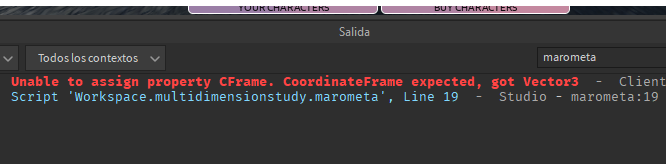# What do I add to this small and simple script [guaranteed solution]

I’m planning that when the player presses a key, a displacement animation is activated and at the same time the position of the main part is changed:

``````local player = game.Players.LocalPlayer
local uis = game:GetService("UserInputService")
local char = player.Character
local animacion = script:WaitForChild("Animation")
local humanoid = char:WaitForChild("Humanoid")
local parteprincipal = char:FindFirstChild("HumanoidRootPart")
local dummy = game.Workspace.Dummy
local value = true

uis.InputBegan:Connect(function(key)
if key.KeyCode == Enum.KeyCode.E then
if value then
value = false
activar:Play()

wait(1)

parteprincipal.CFrame = parteprincipal.CFrame.LookVector +  Vector3.new(0,0,10)
--dummy.HumanoidRootPart.CFrame.LookVector = CFrame.new(Vector3.new(0,0,3))
wait(5)
value = true

end
end
end)
``````

but I get this in the output:What I want most is that if you can change the script so that it works as I said at the beginning of I would thank you very much and a solution

You are trying to set a `Vector3` value to a property that only accepts `CFrame`.

1 Like

I tried it with CFrame but it still gave me the error

The `LookVector` of a `CFrame` is a `Vector3`.
If you’re looking to move the `parteprincipal` instance backwards, try this instead:

``````parteprincipal.CFrame *= CFrame.new(0,0,10)
``````
1 Like

and for the front (that is, the HumanoidRootPart of the player character)
I want to move it to where the player (character) looks

It would be `-10` instead of `10` for moving forward.

You are trying to set the CFrame of the part, but you are giving it a Vector3 (LookVector is a vector3 and Vector3.new(0,0,10) is obviously also a Vector3) Instead, try to set the position instead of the CFrame (parteprincipal.Position = etc)